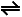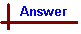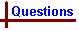Non-ionic equilibrium (Advanced questions) Your feedback on these self-help problems is appreciated. Click here to send an e-mail. Shortcut to Questions Q: 1 2 3 4 5 6 7 8 9 1 Predict, from Le Chateliers's Principle, the effect of: (a) temperature increase at fixed volume and, (b) pressure increase causing reduction in volume, on each of the following equilibria: (1.) 4HCl(g) + O2(g)2Cl2(g) + 2H2O(g) (ΔH is negative) (2.) CO(g) + H2(g)C(s) + H2O(g) (ΔH is negative) (3.) CaCO3(s)CaO(s) + CO2(g) (ΔH is positive) (4.) 2SO2(g) + O2(g)2SO3(g) (ΔH is positive) (5.) Fe3O4(s) + 4H2(g)3Fe(s) + 4H2O(g) (ΔH is negative)2 (1.) Calculate ΔH at 298 K for the forward reaction in the gaseous equilibrium: PCl5(g)PCl3(g) + Cl2(g) (2.) Explain qualitatively the effect upon the above equilibrium as a result of the following separate changes being made: (a) Increased pressure causing reduction in volume (b) Addition of chlorine gas at constant volume (c) Addition of phosphorus pentachloride gas at constant volume (d) Increased temperature at constant volume (e) Presence of a catalyst3 (1.) For the reaction HI(g)½H2(g) + ½I2(g) write the expression for the equilibrium constant, Kc. (2.) At 723 K the value of Kc for the above reaction is 0.141. Calculate the value of the equilibrium constant for the reaction H2(g) + I2(g)2HI(g) at this temperature.4 Acetic acid (1.00 mole) reacts with ethanol (1.00 mole). After esterification at 298 K the equilibrium mixture (total volume 113 mL) is found by titration to contain 0.34 mole of acetic acid. (1.) Calculate the equilibrium constant for this reaction. (2.) The volume is then increased to 1130 mL by addition of diethyl ether (an inert solvent) and the equilibrium re-established at 298 K. What amount of acetic acid is now present?5 When iodine gas (46.0 g) and hydrogen gas (1.00 g) are heated to 450 °C and the equilibrium H2(g) + I2(g)2HI(g) is established, the equilibrium mixture contains iodine gas (1.90 g). Calculate: (1.) The amount (mole) of each gas in the equilibrium mixture (2.) Kp6 In an equilibrium mixture of nitrogen, hydrogen and ammonia gases contained in a 5.00 litre reaction vessel at 450 °C and a total pressure of 332 atmosphere, the partial pressures of the gases were: N2 = 48 atmosphere, H2 =142 atmosphere, NH3 = 142 atmosphere. Calculate Kc for the reaction N2 + 3H22NH3 at 450 °C.7 Kp for the reaction N2(g) + 3H2(g)2NH3(g) is 9.05 x 10-4 at 300 °C. What amount of NH3 was introduced into a 1.00 litre reaction vessel if, at equilibrium at 300 °C, the mixture contained nitrogen gas (2.00 mole)?8 For the reaction CO(g) + H2O(g)CO2(g) + H2(g), Kc at 1259 K is 0.63. A mixture of water vapour (1.00 mole) and carbon monoxide (3.00 mole) at a constant total pressure of 200 kPa is allowed to come to equilibrium at 1259 K. Find: (1.) the amount (mole) of H2 at equilibrium (2.) the total pressure at equilibrium and (3.) the partial pressure of each gas in the equilibrium mixture.9 Pure phosphorus pentachloride gas is introduced into an evacuated vessel and comes to equilibrium at 250 °C, the reaction being PCl5(g)PCl3(g) + Cl2(g). The total pressure is 202 kPa and the mole fraction of the chlorine gas is 0.407. (1.) What are the partial pressures of phosphorus trichloride and phosphorus pentachloride gases? (2.) Calculate Kp for the reaction at 250 °C.Non-ionic equilibrium (Advanced answers) 1 (1.) 4HCl(g) + O2(g)2Cl2(g) + 2H2O(g) (ΔH is negative) (a) The reaction is exothermic, so an increase in temperature will favour formation of reactants. (b) Δn gas is negative (ie there are more moles of gaseous reactants than gaseous products), so an increase in pressure will favour the formation of products. (2.) CO(g) + H2(g)C(s) + H2O(g) (ΔH is negative) (a) Reaction is exothermic, so formation of reactants is favoured. (b) Δn gas is negative, so the formation of products is favoured. (3.) CaCO3(s)CaO(s) + CO2(g) (ΔH is positive) (a) Reaction is endothermic, so formation of products is favoured. (b) Δn gas is positive, so the formation reactants is favoured. (4.) 2SO2(g) + O2(g)2SO3(g) (ΔH is positive) (a) Reaction is endothermic, so formation of products is favoured. (b) Δn gas is negative, so formation of products is favoured. (5.) Fe3O4(s) + 4H2(g)3Fe(s) + 4H2O(g) (ΔH is negative) (a) Reaction is exothermic, so formation of reactants is favoured. (b) There are the same no. mol gas on each side of the reaction, so applying pressure will produce no effect on the equilibrium.2 PCl5(g)PCl3(g) + Cl2(g) (1.) ΔH° = ΔH°[PCl3(g)] + ΔH°[Cl2(g)] - ΔH°[PCl5] = (-287 kJ mol-1) + (0 kJ mol-1) - (-375 kJ mol-1) = +88 kJ mol-1. (2.) (a) Since the products contain more mol gas than the reactant, an increase in pressure will favour formation of reactant. (b) Addition of Cl2 will favour formation of reactant. (c) Adding PCl5(g) will favour formation of products. (d) Since the reaction is endothermic, and increase in temperature will favour formation of products. (e) Addition of a catalyst will not affect the equilibrium concentrations, but simply reduce the time required to reach equilibrium.3 (1.) HI(g)½H2(g) + ½I2(g) Kc1 = [H2]½ [I2]½ / [HI] (Kc = 0.141 at 723 K). (2.) H2(g) + I2(g)2HI(g) Kc2 = [HI]2 / [H2] [I2] By manipulating the terms it can be seen that the expression for Kc2 is equal to the expression for Kc1 inverted and squared, ie [HI]2 / [H2] [I2] = ( [H2]½ [I2]½ / [HI] ) -2. It follows then that Kc2 = (Kc1) -2 = 0.141 -2 = 50.3.4 Balanced equation: CH3COOH + CH3CH2OHCH3COOCH2CH3 + H2O (1.) Kc = [CH3COOCH2CH3] [H2O] / [CH3COOH] [CH3CH2OH] If the reaction mixture contains 0.34 mol CH3COOH at equilibrium, it must also contain 0.34 mol CH3CH2OH. This implies that (1.00 mol - 0.34 mol) = 0.66 mol CH3COOH and CH3CH2OH have reacted to form 0.66 mol CH3COOCH2CH3 and 0.66 mol H2O. These values now need to be converted into concentrations. The question specifies a reaction volume of 0.113 L, hence obtaining the following equilibrium concentrations: [CH3COOH] = 3.009 mol L-1 [CH3CH2OH] = 3.009 mol L-1 [CH3COOCH2CH3] = 5.841 mol L-1 [H2O] = 5.841 mol L-1 Having inferred the concentrations of all species at equilibrium, Kc can be determined from the expression given above: Kc = (5.841 mol L-1) (5.841 mol L-1) / (3.009 mol L-1) (3.009 mol L-1) = 3.8. (2.) Kc = [CH3COOCH2CH3] [H2O] / [CH3COOH] [CH3CH2OH] KC will remain constant. Upon addition of inert solvent the concentration of all species is reduced to 1/10, but as there are equal amounts of reactants and products in the equilibrium expression, these changes effectively cancel each other out, and the amount of each component remains constant.5 H2(g) + I2(g)2HI(g) (1.) Amount of H2 before equilibration = 1.00 g = 0.496 mol. Amount of I2 before equilibration = 46.0 g = 0.181 mol Amount of I2 at equilibrium = 1.90 g = 0.00749 mol. The no. mol H2 at equilibrium = no. mol I2 (ie for each mole of unreacted I2 there must also be a mole of unreacted H2) + stoichiometric excess H2 (in this reaction H2 is in excess, so even if reaction went to completion there would be H2 present in the reaction mixture).∴ No. mol H2 at equilibrium = 0.00749 mol. + (0.496 mol - 0.181 mol) = 0.322 mol Each mole of I2 which reacts produces two moles HI. No. mol I2 converted to HI = (0.181 mol - 0.00749 mol) = 0.1735 mol. ∴ No. mol HI formed = 2 x 0.1735 mol = 0.347 mol. (2.) Kp = p2HI / pH2 pI2 Kp = Kc in this example since Δn gas = 0. The units in the expression for K cancel, so we can use the no. mol each species directly, without converting into concentration. ∴ Kc = Kp = (0.348 mol HI)2 / (0.00749 mol I2) (0.322 mol H2) = 50.2.6 Total pressure = 332 atm pNH3 = 142 atm pH2 = 142 atm pN2 = 48 atm First we find Kp, and then Kc: Kp = p2NH3 / pN2 p3H2 = 1422 / 48 x 1423 = 1.47 x 10-4 atm-2 Now, T = 723 K, R = 0.0821 L atm K-1 mol-1, Δn gas = -2. From Kc = Kp(RT) -Δn gas: Kc = 1.47 x 10-4 atm-2 (0.0821 L atm K-1 mol-1 x 723 K) 2 = 1.47 x 10-4 x 3523 L2 mol-2 = 0.52 L2 mol-2.7 N2(g) + 3H2(g)2NH3 (Kp 9.05 x 10-4 atm-2 at 573 K) [N2] = 2.00 mol L-1 ∴[H2] = 6.00 mol L-1 We can calculate [NH3] by converting Kp into Kc and solving for [NH3]: Δn gas = -2, R = 0.0821 L atm K-1 mol-1, T = 573 K: Kc = Kp(RT) -Δn gas = 9.05 x 104 atm-2 (0.0821 L atm K-1 mol-1 x 573 K)2 = 2.00 L2 mol-2. Now Kc = [NH3]2 / [N2] [H2]3. ∴[NH3] = (Kc [N2] [H2]3)½ = (2.00 L2 mol-2 x 2.00 mol L-1 x 6.003 mol3 L-3)½ = (864 mol2 L-2)½ = 29.4 mol L-1 at equilibrium. Amount of NH3 before equilibrium = amount of NH3 at eqquilibrium + amount of NH3 converted into N2 and H2 = 29.4 mol L-1 + 4.00 mol L-1 = 33.4 mol L-1. Volume of reaction vessel = 1.00 L,∴Amount of NH3 present before equilibrium = 33.4 mol.8 CO(g) + H2O(g)CO2(g) + H2(g) (Kc = 0.63 at 1259 K) (1.) Kc = [CO2] [H2] / [CO] [H2O]. Kc is unitless, so species' concentrations in the expression for Kc can be replaced by the number of mol species, since the units cancel out. Let x = no. mol H2 = no. mol CO2. Then Kc = 0.63 = x2 / (1 - x)(3 - x). From this we obtain the quadratic: -0.37x2 - 2.52x + 1.89 = 0. Using x = ( -b ± (b2 - 4ac)½ ) / 2a we obtain x = -7.486 or 0.676. We select 0.676 since -7.486 holds no significance in this problem. ∴ no. mol H2 = 0.676 mol. (2.) The pressure at equilibrium is the same as before reaction since the total number of moles of gas is unchanged (ie Δn gas = 0). ∴ P = 200 kPa at equilibrium. (3.) Partial pressure is proportional to the number of moles of gas present (at constant V, T, from n = PV / RT). Total pressure = 200 kPa, total no. mol gas = 4.00 mol. No. mol H2O at equilibrium = 1 - x = 0.324 mol No. mol CO at equilibrium = 3 - x = 2.324 mol No. mol H2 at equilibrium = x = 0.676 mol No. mol CO2 at equilibrium = x = 0.676 mol Mole fraction H2O = 0.324 mol / 4.00 mol = 0.0810. Partial pressure H2O = mole fraction H2O x total pressure = 0.0810 x 200 kPa = 16.2 kPa. Mole fraction CO = 2.324 mol / 4.00 mol = 0.581. Partial pressure CO = mole fraction CO x total pressure = 0.581 x 200 kPa = 116 kPa. Mole fraction H2 = 0.676 mol / 4.00 mol = 0.169. Partial pressure H2 = mole fraction H2 x total pressure = 0.169 x 200 kPa = 33.8 kPa. Mole fraction CO2 = 0.676 mol / 4.00 mol = 0.169. Partial pressure CO2 = mole fraction CO2 x total pressure = 0.169 x 200 kPa = 33.8 kPa.9 PCl5(g)PCl3(g) + Cl2(g) (1.) Mole fraction Cl2 = mole fraction PCl3 = 0.407. Mole fraction PCl5 = 1 - (mole fraction Cl2 + mole fraction PCl3) = 1 - (2 x 0.407) = 0.186. Total pressure = 202 kPa pPCl3 = 0.407 x 202 kPa = 82.2 kPa pPCl5 = 0.186 x 202 kPa = 37.6 kPa (2.) Kp can be calculated from the partial pressures, after converting them into atmospheres (1 atm = 101.3 kPa ): pPCl3 = 0.812 atm pPCl5 = 0.371 atm pCl2 = 0.812 atm Kp = pPCl3 pCl2 / pPCl5 = 0.812 atm x 0.812 atm / 0.371 atm = 1.78 atm.